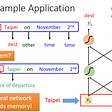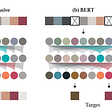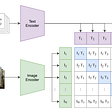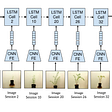# TensorFlow 1.0 vs 2.0, Part 1: Computational Graphs

TensorFlow, a machine learning library created by Google, is not known for being easy to use. In response, TensorFlow 2.0 addressed a lot of the pain points with eager mode and AutoGraph features. Thing is, while these additions solve a lot of problems, they also complicate the existing programming model.

In this article, I’ll introduce the TensorFlow 1.0 programming model and discuss some of the design choices and accompanying problems. This will make the updates in TensorFlow 2.0, which we’ll discuss in the next part of the series, easier to understand.

# Core Concepts

The TensorFlow framework has two components:

• Library: for defining computational graphs.
• Runtime: for executing such graphs on a variety of different hardware platforms.

If you want to learn more, check out the official documentation on TensorFlow architecture.

If you are building your machine learning algorithms with TensorFlow, all the work you are doing is translating ML model designs into TensorFlow computational graphs and submitting them to the runtime. Then as always, the runtime takes care of the rest.

# What Are Computational Graphs?

In TensorFlow, machine learning algorithms are represented as computational graphs. A computational graph is a type of directed graph where nodes describe operations, while edges represent the data (tensor) flowing between those operations.

Before we dig deeper, let’s learn the building blocks of the graphs.

• Tensors: A tensor is a description of a multidimensional array. Tensors may have a shape and data type, but don’t have actual values. Tensor shapes can usually be derived from the graph.
• Operations: An operation can have zero or more inputs and produce zero or more outputs. As such, an operation may represent a mathematical equation, variable, constant, or a control flow directive.
• Variables: All trainable parameters of machine learning models are tf.Variables. A variable is defined by its name, type, shape, and initialization procedure.

The words “Tensor” and “Flow” mean that tensors (data) flow through the computational graph. Computational graphs are not specific to TensorFlow — PyTorch and other machine learning frameworks use them as well.

Here are some advantages of using computational graphs:

• Dependency driven scheduling. Namely the data dependencies, which specify the order of execution. Operations that do not depend on one another can be scheduled in parallel.
• Graph Optimizations. Such as common subgraph elimination.
• Automatic Differentiation. Describing our computations as graphs allow us to easily compute gradients. If we know the gradients of each operation’s output with respect to its direct inputs, the chain rule provides us with gradients for any Tensor with respect to any other. This process is known as reverse mode auto-differentiation, and it enables the computation of a node’s gradient in the graph with respect to all others in a single sweep.

# Computational Graphs in Action

When we define the graph, we make use of a set of TensorFlow library functions to specify computations as a tf.Graph. At execution time, we use the TensorFlow runtime to execute those computations through a tf.Session.

tf.Session carries out the actual computations; session.run executes the graph and returns the value of the tensor. When session.run() is called, TensorFlow identifies and executes the smallest set of nodes that needs to be evaluated in order to compute the requested tensors.

To see a graph in action, let’s build a tf.Graph:

`a = tf.constant(1.0) #  this is an op and adds a node to the default tf.Graphb = tf.constant(1.0)c = tf.add(a,b)with tf.Session() as sess:  print(sess.run(c))`

This is the simplest graph we can build. But we have not mentioned the graph once, and without explicit specification, all the operations (nodes) will be added to the global tf.Graph instance.

In my opinion, because this API behavior can be a bit confusing for beginners, I believe tf.Graph should not be hidden from the user. However, we can create our tf.Graph instance (recommended) and limit our operations to it.

We can refactor the code sample above with explicit graph definition as such:

`g1 = tf.Graph()with g1.as_default() as g:  a = tf.constant(1.0)  b = tf.constant(1.0)  c = tf.add(a,b)with tf.Session(graph = g1) as sess:  print(sess.run(c))`

# Variables in TensorFlow 1.0

Among all aspects of TensorFlow 1.0, the variables are the trickiest. Here are a few things to understand about tf.Variables:

• A variable instance exists and holds values in the context of a specific session.
• Any variable we define must be explicitly initialized before its first use in a session. A quick-fix, although perhaps a bit lazy, is to initialize all the variables using tf.global_variables_initializer().

# Data Injection With Placeholders

Now that we’ve learned how to build the graph, how can we feed in the data? This is where placeholders come into play.

`a = tf.placeholders(tf.float32, [])b = tf.constant(1.0)c = tf.add(a,b)with tf.Session() as session:  print(session.run(c, feed_dict={a:1.0}))  print(session.run(c, feed_dict={a:2.0}))`

We can use placeholders and feed dictionaries to inject data into the graph at execution time. Placeholders are used in the graph as tensors but at each execution of the graph, they’ll take the value specified in the feed dictionary provided to session.run.

It’s obvious that the placeholder variable and key in the feed_dict should be the same, and that is why we use the term data injection.

# Control Dependency

Dealing with graphs is a bit different from using a pure Pythonic way of programming. For example:

`a = tf.constant(1.0)b = tf.Variable(2.0)assign_b = tf.assign(b, 10)c = tf.add(a,b)with tf.Session() as sess:  sess.run(tf.global_variables_initializer())  print(sess.run(c))`

You might have expected the output of c to be 11, but it prints 3. When there is a dependency between operations, especially a hidden dependency — such as the one between the c and assign_b operations, we have to explicitly declare such relationships.

To fix the problem, we can change the code as follows:

`a = tf.constant(1.0)b = tf.Variable(2.0)assign_b = tf.assign(b, 10)with tf.control_dependencies([assign_b]):  c = tf.add(a,b)with tf.Session() as sess:  sess.run(tf.global_variables_initializer())  print(sess.run(c))`

And now we can see that the output is 11, as desired.

# In Summary

TensorFlow with the computational graphs might not be as intuitive as other languages or frameworks but you’ll find the graphs to be powerful and performant in practice.

With eager mode in TensorFlow 2.0 knocking at the door, I still believe computational graphs are here to stay for many, many days to come. :)

Thanks for reading! If you enjoyed this article, please hit the clap button below. It would mean a lot and encourage me to write more like it. Stay tuned for the next part in the series! :)

Questions or comments? I’d love to hear from you!

--

--

--

## More from AI³ | Theory, Practice, Business

The AI revolution is here! Navigate the ever changing industry with our thoughtfully written articles whether your a researcher, engineer, or entrepreneur

## Note: Recurrent Neural Network (RNN)## Review — iGPT: Generative Pretraining from Pixels## Why Linear Regression is not suitable for Binary Classification## Filtering Noises in Covarience Matrix## Increasing Reliance on “Advanced Algorithms”## Apple Silicon Mac M1 natively supports TensorFlow 2.6## Testing and Monitoring Machine Learning Model Deployments## OpenCv python: solve sudoku from image## Yusup

http://blog.lsgrep.com

## Having fun with CLIP features — Part I## Synopsis: Intelligent Monitoring of Stress Induced by Water Deficiency in Plants Using Deep…